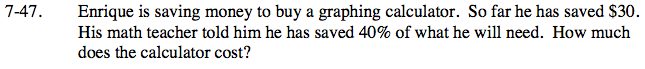Home > ACC7 > Chapter cc27 > Lesson cc27.1.4 > Problem7-47

7-47.

Enrique is saving money to buy a graphing calculator. So far he has saved $30. His math teacher told him he has saved 40% of what he will need. How much does the calculator cost? Homework Help ✎If 30 dollars is 40% of the total amount Enrique needs, what is the total amount of money Enrique needs?$30 = 0.40(total price)

\$75